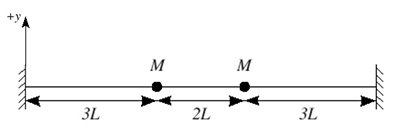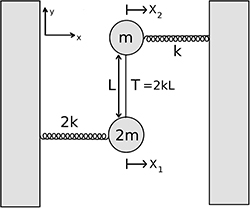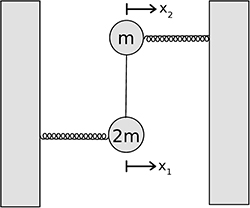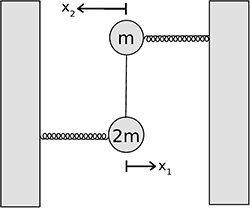RES.8-005 | Fall 2012 | Undergraduate

# Vibrations and Waves Problem Solving

Coupled Oscillators without Damping

## Problems

### Coupled Oscillators without Damping

#### Problem 1

There are two small massive beads, each of mass $$M$$, on a taut massless string of length $$8L$$, as shown. The tension in the string is $$T$$.1. Derive expressions for the normal mode angular frequencies of the system, for small displacements of the system in a plane. Assume that $$T$$ is sufficiently large that you may ignore gravity.
2. If at $$t = 0$$ both masses are stationary, one is at equilibrium and the other displaced from equilibrium by a distance $$A$$, write an expression for the displacement $$y_{1}(t)$$ as a function of time of the mass initially in the equilibrium position.

1. The two normal mode angular frequencies are $$\hspace{1mm} \omega_1 = \sqrt{\dfrac{T}{3LM}}\hspace{2mm} and \hspace{2mm} \omega_2 = \sqrt{\dfrac{4T}{3LM}}$$.
2. \begin{eqnarray} \nonumber y_1(t) &=& \frac{A}{2}\cos \omega_1 t - \frac{A}{2}\cos \omega_2 t \\ \nonumber &=& \frac{A}{2}\cos \left (\sqrt{\frac{T}{3LM}} t\right ) - \frac{A}{2}\cos \left (\sqrt{\frac{4T}{3LM}} t\right ) \end{eqnarray}

#### Problem 2The figure above shows a system of masses in the x-y plane. The mass of $$2m$$ is connected to an immobile wall with a spring of constant $$2k$$, while the mass of $$m$$ is connected to an immobile wall with a spring of constant $$k$$. The masses are then coupled to each other with an elastic band of length $$L$$, under tension $$T=2kL$$. The masses are constrained to move in the $$x$$ direction only. At equilbrium the masses have the same $$x$$ position and the springs are uncompressed. There is no friction or gravity. The displacements from equilibrium are small enough ($$x_1, x_2 \ll L$$), so that the tension in the band stays constant.

1. Find the normal mode frequencies of the system.
2. On the two figures included on the next page sketch the normal modes of the system, be sure to clearly indicate both the magnitude and direction of the motion of the masses.

1. The two normal mode frequencies are $$\hspace{1mm} \omega_1 = \sqrt{\frac{k}{m}} \hspace{2mm} and \hspace{2mm} \omega_2 = 2\sqrt{\frac{k}{m}}\hspace{1mm} .$$